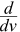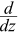# Derivative Rules

The Derivative tells us the slope of a function at any point.There are rules we can follow to find many derivatives.

For example:

• The slope of a constant value (like 3) is always 0
• The slope of a line like 2x is 2, or 3x is 3 etc
• and so on.

Here are useful rules to help you work out the derivatives of many functions (with examples below). Note: the little mark means "Derivative of", and f and g are functions.

Common Functions Function
Derivative
Constant c 0
Line x 1
ax a
Square x2 2x
Square Root √x (½)x
Exponential ex ex
ax ln(a) ax
Logarithms ln(x) 1/x
loga(x) 1 / (x ln(a))
Trigonometry (x is in radians) sin(x) cos(x)
cos(x) −sin(x)
tan(x) sec2(x)
Inverse Trigonometry sin-1(x) 1/√(1−x2)
cos-1(x) −1/√(1−x2)
tan-1(x) 1/(1+x2)

Rules Function
Derivative
Multiplication by constant cf cf’
Power Rule xn nxn−1
Sum Rule f + g f’ + g’
Difference Rule f - g f’ − g’
Product Rule fg f g’ + f’ g
Quotient Rule f/g (f’ g − g’ f )/g2
Reciprocal Rule 1/f −f’/f2

Chain Rule
(as "Composition of Functions")
f º g (f’ º g) × g’
Chain Rule (using ’ ) f(g(x)) f’(g(x))g’(x)
Chain Rule (using d dx ) dy dx = dy du du dx

"The derivative of" is also written d dx

So d dx sin(x) and sin(x)’ both mean "The derivative of sin(x)"

## Examples

### Example: what is the derivative of sin(x) ?

From the table above it is listed as being cos(x)

It can be written as:sin(x) = cos(x)

Or:

sin(x)’ = cos(x)

### Example: What isx3 ?

The question is asking "what is the derivative of x3 ?"

We can use the Power Rule, where n=3:xn = nxn−1x3 = 3x3−1 = 3x2

(In other words the derivative of x3 is 3x2)

So it is simply this:"multiply by power
then reduce power by 1"

It can also be used in cases like this:

### Example: What is(1/x) ?

1/x is also x-1

We can use the Power Rule, where n = −1:xn = nxn−1x−1 = −1x−1−1 = −x−2

So we just did this:which simplifies to −x−2

### Example: What is5x3 ?

the derivative of cf = cf’

the derivative of 5f = 5f’

We know (from the Power Rule):x3 = 3x3−1 = 3x2

So:5x3 = 5x3 = 5 × 3x2 = 15x2

### Example: What is the derivative of x2+x3 ?

The Sum Rule says:

the derivative of f + g = f’ + g’

So we can work out each derivative separately and then add them.

Using the Power Rule:

•x2 = 2x
•x3 = 3x2

And so:

the derivative of x2 + x3 = 2x + 3x2

### Difference Rule

It doesn't have to be x, we can differentiate with respect to, for example, v:

### Example: What is(v3−v4) ?

The Difference Rule says

the derivative of f − g = f’ − g’

So we can work out each derivative separately and then subtract them.

Using the Power Rule:

•v3 = 3v2
•v4 = 4v3

And so:

the derivative of v3 − v4 = 3v2 − 4v3

### Example: What is(5z2 + z3 − 7z4) ?

Using the Power Rule:

•z2 = 2z
•z3 = 3z2
•z4 = 4z3

And so:(5z2 + z3 − 7z4) = 5 × 2z + 3z2 − 7 × 4z3 = 10z + 3z2 − 28z3

### Example: What is the derivative of cos(x)sin(x) ?

The Product Rule says:

the derivative of fg = f g’ + f’ g

In our case:

• f = cos
• g = sin

We know (from the table above):

•cos(x) = −sin(x)
•sin(x) = cos(x)

So:

the derivative of cos(x)sin(x) = cos(x)cos(x) − sin(x)sin(x)

= cos2(x) − sin2(x)

### Quotient Rule

(fg)’ = gf’ − fg’g2

The derivative of "High over Low" is:

"Low dHigh minus High dLow, over the line and square the Low"

### Example: What is the derivative of cos(x)/x ?

In our case:

• f = cos
• g = x

We know (from the table above):

• f' = −sin(x)
• g' = 1

So:

the derivative of cos(x)x = Low dHigh minus High dLowover the line and square the Low

= x(−sin(x)) − cos(x)(1)x2

= −xsin(x) + cos(x)x2

### Example: What is(1/x) ?

The Reciprocal Rule says:

the derivative of 1/f = −f’/f2

With f(x)= x, we know that f’(x) = 1

So:

the derivative of 1/x = −1/x2

Which is the same result we got above using the Power Rule.

### Example: What is d dx sin(x2) ?

sin(x2) is made up of sin() and x2:

• f(g) = sin(g)
• g(x) = x2

The Chain Rule says:

the derivative of f(g(x)) = f'(g(x))g'(x)

The individual derivatives are:

• f'(g) = cos(g)
• g'(x) = 2x

So:

d dx sin(x2) = cos(g(x)) (2x)

= 2x cos(x2)

Another way of writing the Chain Rule is: dy dx = dy du du dx

Let's do the previous example again using that formula:

### Example: What is d dx sin(x2) ?

dy dx = dy du du dx

Have u = x2, so y = sin(u):

d dx sin(x2) = d du sin(u) d dx x2

Differentiate each:

d dx sin(x2) = cos(u) (2x)

Substitue back u = x2 and simplify:

d dx sin(x2) = 2x cos(x2)

Same result as before (thank goodness!)

Another couple of examples of the Chain Rule:

### Example: What is(1/cos(x)) ?

1/cos(x) is made up of 1/g and cos():

• f(g) = 1/g
• g(x) = cos(x)

The Chain Rule says:

the derivative of f(g(x)) = f’(g(x))g’(x)

The individual derivatives are:

• f'(g) = −1/(g2)
• g'(x) = −sin(x)

So:

(1/cos(x))’ = −1/(g(x))2 × −sin(x)

= sin(x)/cos2(x)

Note: sin(x)/cos2(x) is also tan(x)/cos(x), or many other forms.

### Example: What is(5x−2)3 ?

The Chain Rule says:

the derivative of f(g(x)) = f’(g(x))g’(x)

(5x-2)3 is made up of g3 and 5x-2:

• f(g) = g3
• g(x) = 5x−2

The individual derivatives are:

• f'(g) = 3g2 (by the Power Rule)
• g'(x) = 5

So:(5x−2)3 = 3g(x)2 × 5 = 15(5x−2)2